# SPSS for the Classroom: Statistics and Graphs

This document is intended for students taking classes that use SPSS Statistics. Those who plan on doing more involved research projects using SPSS should attend our workshop series.

If you are not already familiar with the SPSS windows (the Data Editor, Output Viewer, and Syntax Editor), please read SPSS for the Classroom: The Basics.

The examples that follow are based on the sample data in C:\Program Files\IBM\SPSS\Statistics\23\Samples\English\Employee data.sav

## Frequencies: Counts and Percents

Counts and percents are wonderful statistics because they are easy to explain and quickly grasped. Frequencies also form the very foundation of most explanations of probability. They are an excellent place to begin understanding any data you may work with.

### Syntax:

frequencies variables = gender minority.

### Output: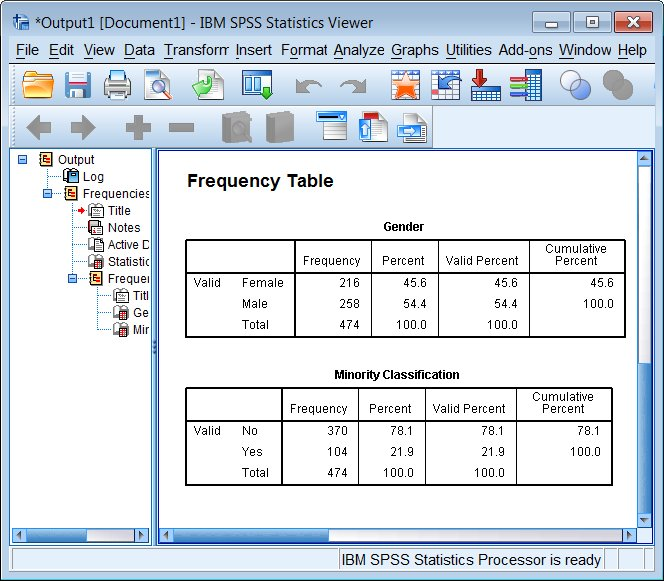Note that this is one of the few tables where missing values (whether system missing "." or user designated missing) show up in the default output table (however, not in this particular example).

## Descriptives: Means and Standard Deviations

The mean and standard deviation of a variable are such fundamental quantities in statistics, that there are many SPSS commands that will report them to you. The most straightforward command to use is Descriptives.

Two other useful commands are Frequencies (in the dialog box, click on the Statistics button), when you want to see counts as well as means and standard deviations (perhaps for Likert scales), and Explore, which gives you such additional statistics as the median and interquartile range as well as a variety of graphs.

### Syntax:

descriptives variables=educ salary.

### Output: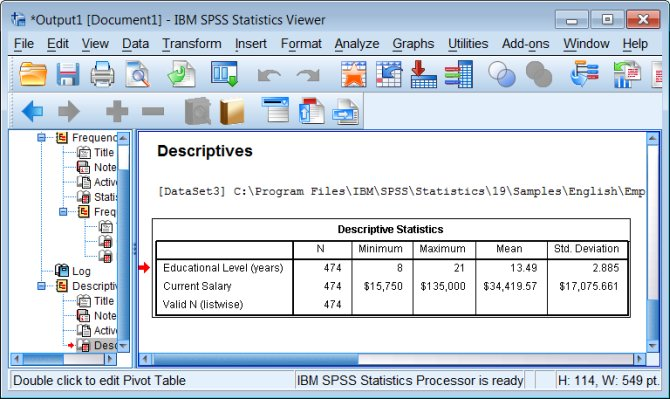## Histograms

SPSS has three different sets of commands for producing graphs. The easiest to learn and use are the oldest "legacy" graphing commands. They give you graphs with a default visual style (colors used, weight of lines, size of type, etc) that can be customized by hand.

Histograms are vexing because they can be alternately informative or deceptive, depending upon how the bins (the bar boundaries) are chosen. They are useful and popular because they are conceptually very simple, easy to draw and interpret, and if drawn well they can give a good visual representation of the distribution of values of a variable.

### Syntax:

graph /histogram(normal) = prevexp.

### Output:In this example, the distribution of the data is nothing like a normal distribution!

To edit colors, titles, scales, etc. double-click on the graph in the Output Viewer, then double-click on the graph element you want to change.

## Crosstabs: Counts by Group

The basic crosstabs command just gives you counts by default. Typically it is useful to also look at either row-percents or column-percents, which must be specified as options.

### Syntax:

crosstabs
/tables=jobcat by minority
/cells=count row.

In this command syntax (and the next one, MEANS), you see the key word BY used to specify a categorical variable that divides the data into groups.

### Output: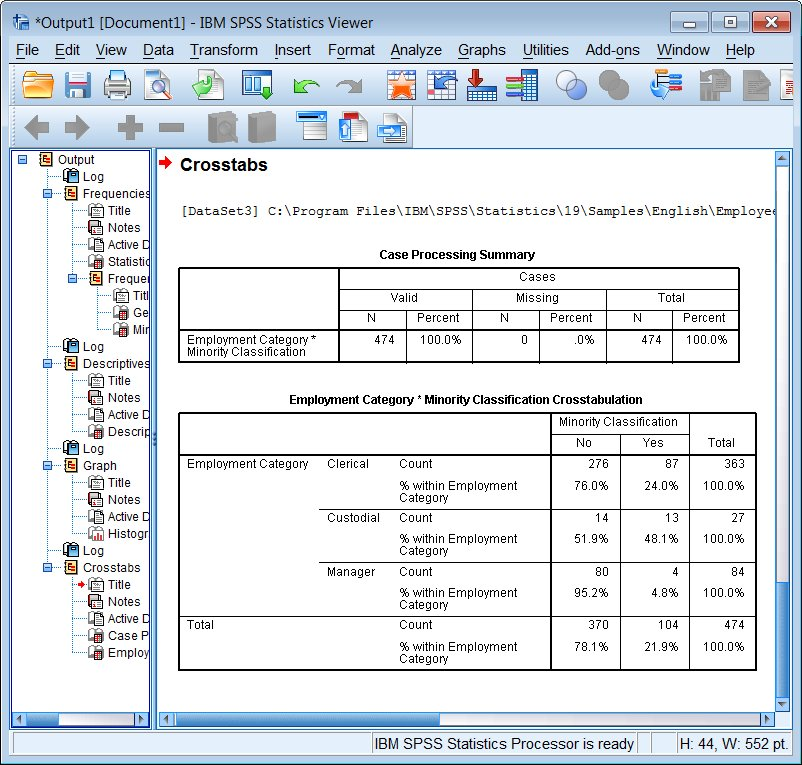## Means by Group

### Syntax:

means tables=salary by minority.

### Output: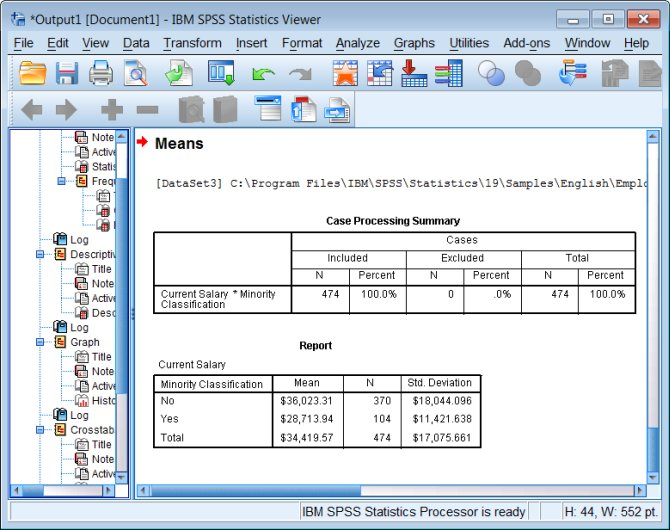## Bar Charts

Similar to a histogram, the x axis is treated as a categorical variable, and the y axis represents one of a variety of summary statistics: counts (a.k.a. a histogram!), means, sums, etc.

### Syntax:

graph /bar=mean(salary) by jobcat.

### Output: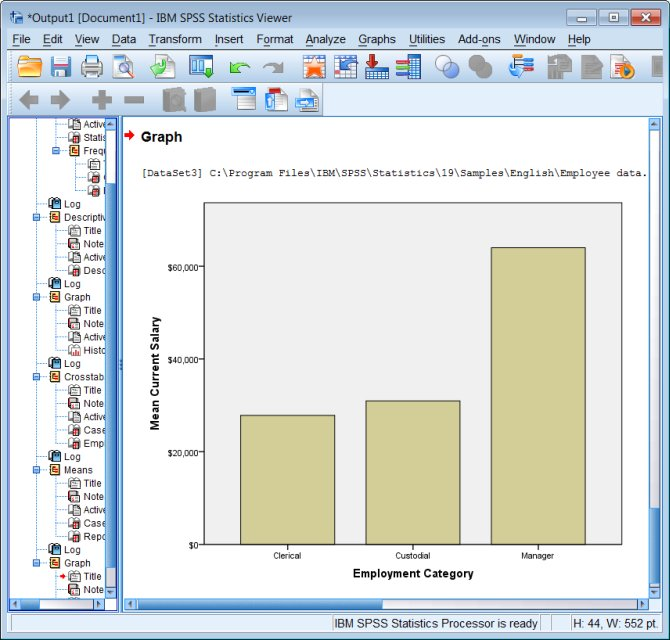## Boxplots

### Syntax:

examine variables=salary by jobcat
/plot=boxplot
/statistics=none
/nototal.

### Output: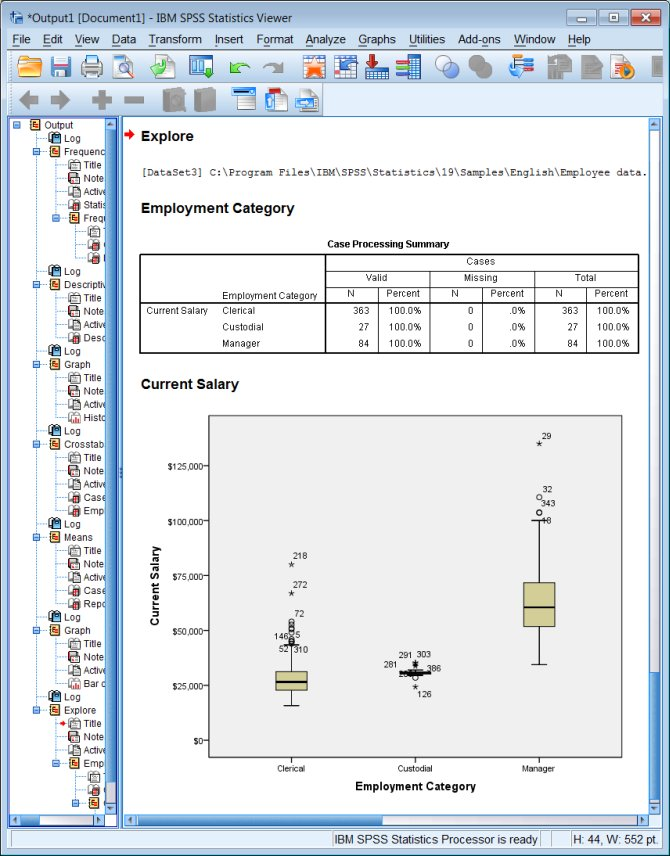## Correlation

### Syntax:

correlations /variables=educ salary prevexp.

### Output:## Scatter Plots

Both simple scatter plots and scatter plot matrixes are pretty easy to produce.

### Syntax:

graph /scatterplot=salary with salbegin.

graph /scatterplot(matrix)=salary salbegin prevexp.

### Output: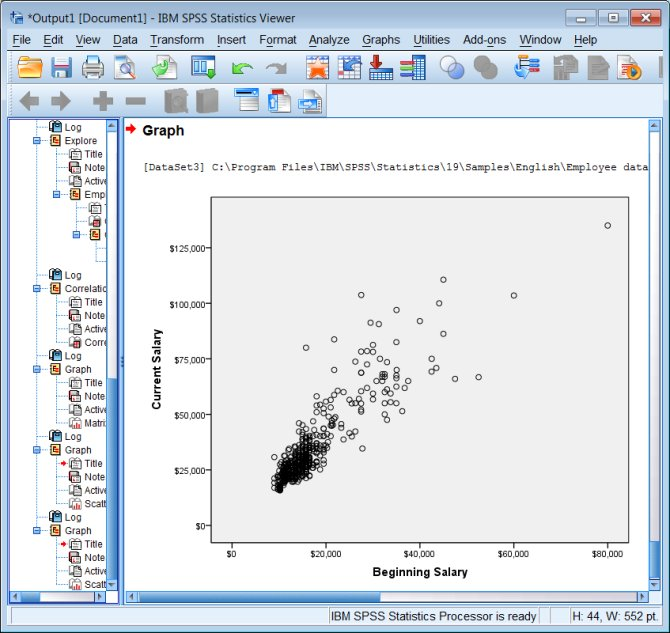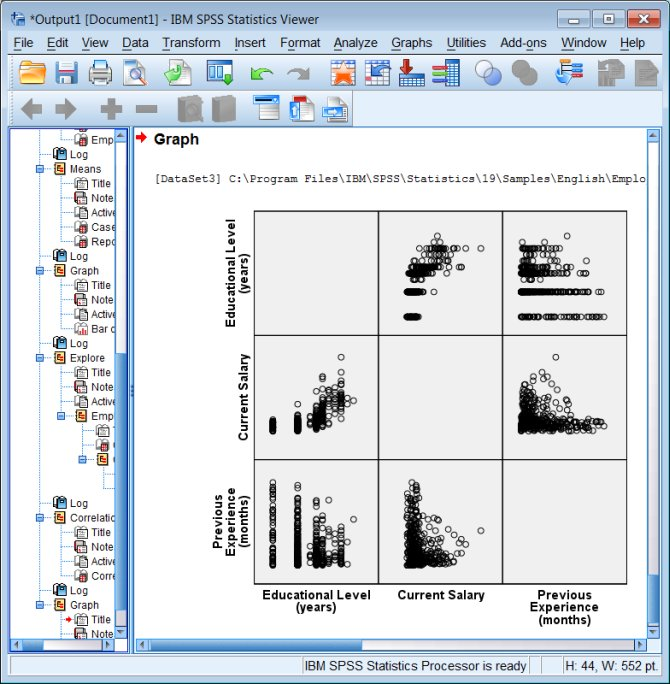## T-tests

T-test can be used in a variety of ways, and SPSS gives you quick access to three of them (univariate, grouped, and paired) through the Compare Means menu. They all access the same t-test command.

### Syntax:

t-test groups=gender('f' 'm')
/variables=educ.

### Output: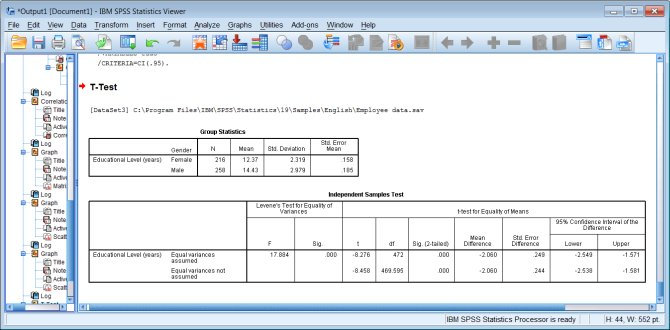## Chi-square Tests

Like t-tests, chi-square tests come up in a wide variety of circumstances, the most common of which is assessing the independence of two variables in a contingency table (a crosstab). So this chi-square test is specified as an option on a crosstab command.

### Syntax:

crosstabs
/tables=jobcat by minority
/statistics=chisq
/cells=count row.

### Output:## ANOVA Tables and Tests

ANOVA tables are a core concept in statistics, and they are produced by several different commands in SPSS, including ONEWAY, GLM, and UNIANOVA. The UNIANOVA command is perhaps the easiest to use overall, because it allows you to use string (character) variables as factors.

(If you are doing a one-way ANOVA and your factor is coded in numeric form, then ONEWAY is even easier to use.)

### Syntax:

unianova salary by jobcat.

### Output: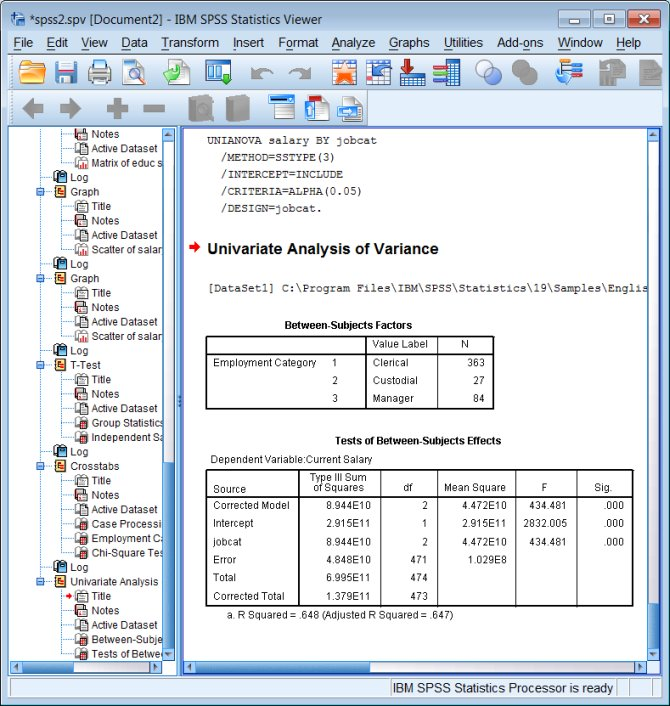## Regression

### Syntax:

regression
/dependent salary
/method=enter salbegin.

### Output:## Learning More

To learn more about how to use the SPSS windows, you can look at the on-line tutorial that comes with the software: click Help - Tutorial.

To learn more about specific data management or statistical tasks, you should try the on-line Help files. Click Help - Topics and you can read about a variety of basic SPSS topics, or search the index.